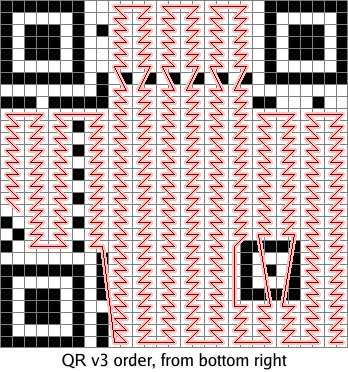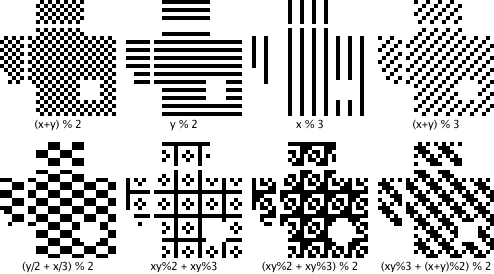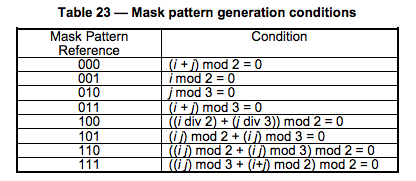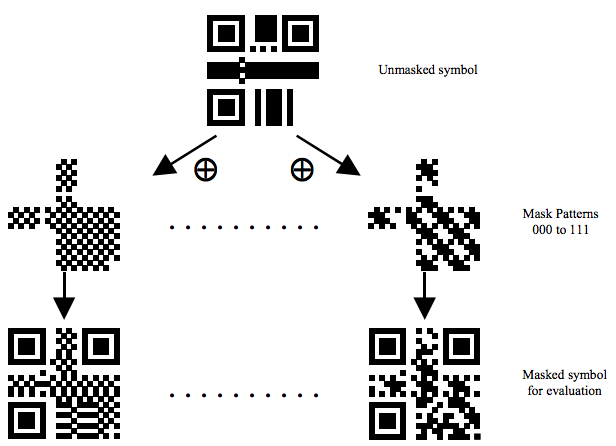# Drawing a QR code

### Position Detection Pattern

First, first draw the Position Detection pattern on three corners. (No matter what the Version, the size of this pattern is so big)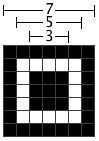### Alignment Pattern

Then, draw the Alignment pattern (regardless of Version, the size of this pattern is so large)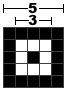For the location of the Alignment, you can view the Table-E.1 definition table on page 81 of the QR Code Spec (the table below is an incomplete table)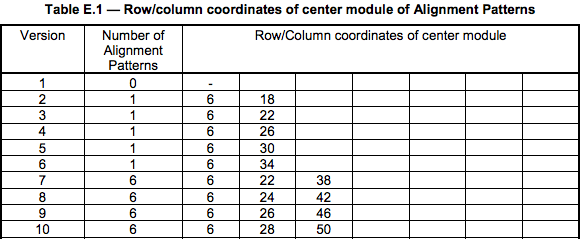The figure below is an example of Version8 in the above table (6, 24, 42)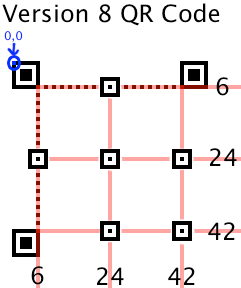### Timing Pattern

Next is the line of Timing Pattern (this needless to say)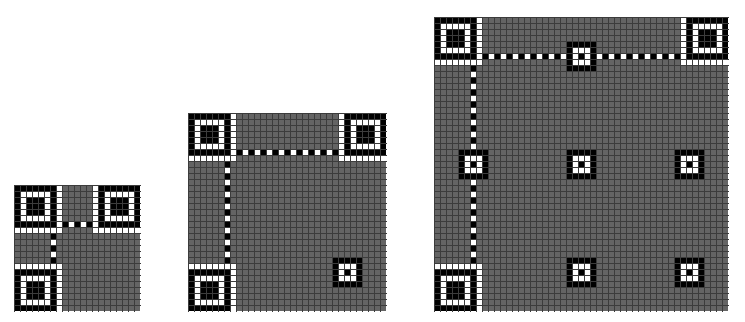### Format Information

Next is the Formation Information, the blue part of the image below.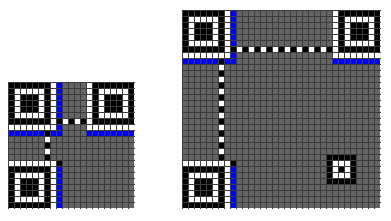Format Information is a 15-bit message, the position of each bit is as shown below: (note the Dark Module in the picture, that is always present)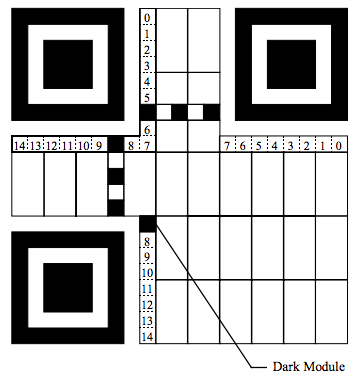These 15 bits include:
• 5 data bits: 2 bits are used to indicate what kind of Error Correction Level is used, and 3 bits are used to indicate which Mask is used.
• 10 error correction bits. Mainly calculated by BCH Code

Then 15 bits will be XORed with 101010000010010. This guarantees that we will not be all white because we have chosen 00 error correction level and 000, which will increase the difficulty of image recognition of our scanner.

Here's an example: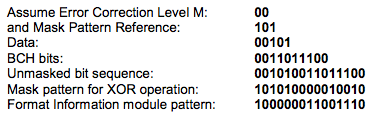The Error Correction Level is shown in the following table: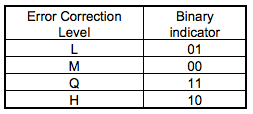The Mask pattern is shown in Table 23 below.

### Version Information

Then there is Version Information (this code is required after version 7), the blue part in the figure below.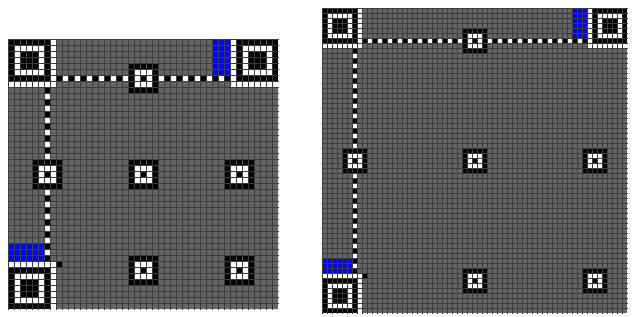Version Information is a total of 18 bits, including the 6-bit version number and 12-bit error correction code. Here is an example: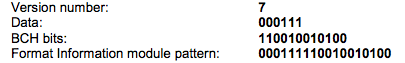And its filling position is as follows: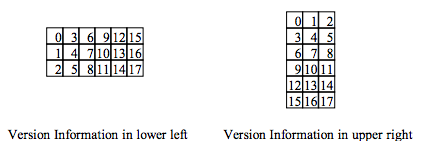### Data and data error correction code

Then fill in our final code, the final code is filled as follows: fill our bits along the red line from the lower left corner, 1 is black, 0 is white. If you encounter the above non-data area, bypass or skip.